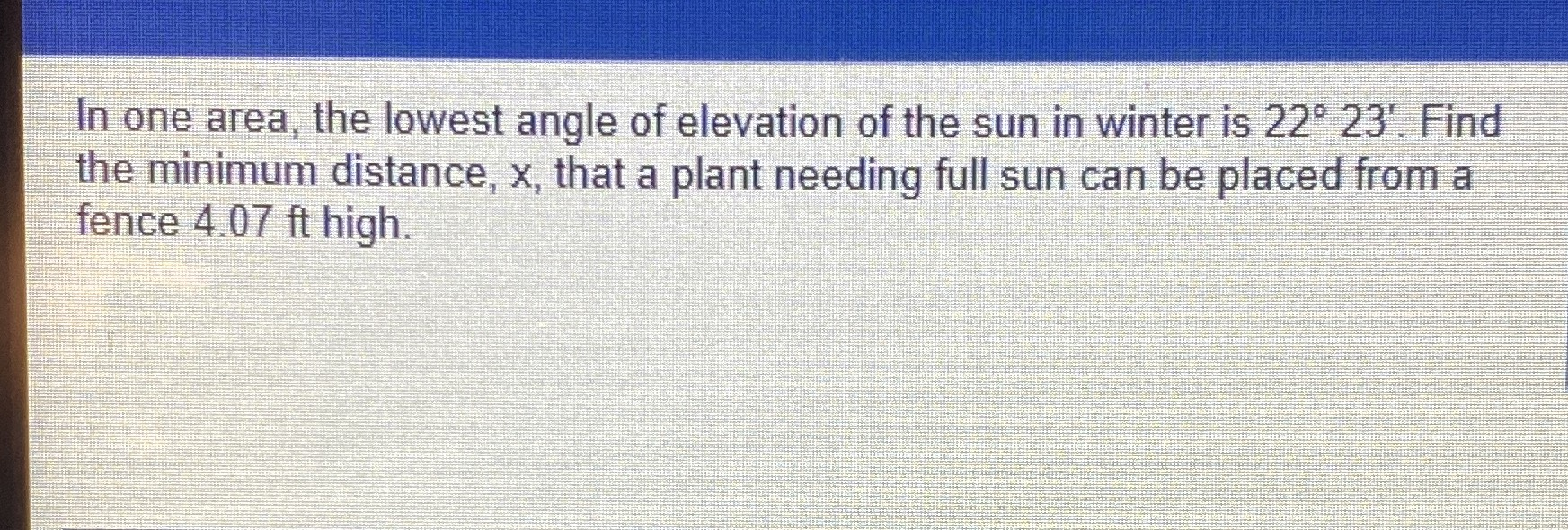### Still have math questions?In one area, the lowest angle of elevation of the sun in winter is $$22 ^ { \circ } 23 ^ { \prime }$$ . Find the minimum distance, $$x$$ , that a plant needing full sun can be placed from a fence $$4.07 ft$$ high  .
$$\frac{4.07}{\sin\left(22\ .38\right)}$$=10.68949542